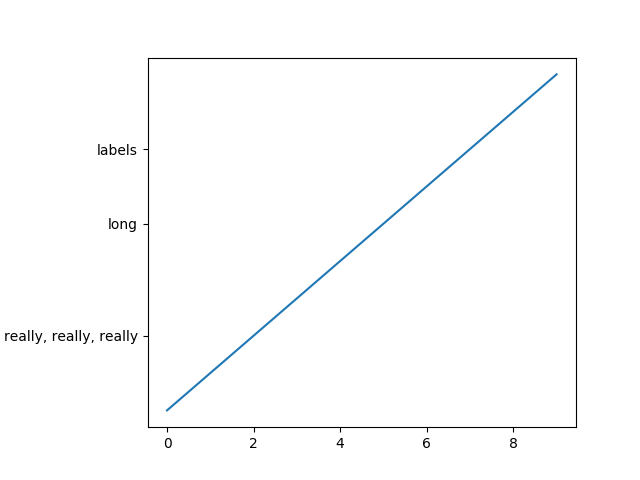### Related Topicsimport matplotlib.pyplot as plt
import matplotlib.transforms as mtransforms
fig, ax = plt.subplots()
ax.plot(range(10))
ax.set_yticks((2,5,7))
labels = ax.set_yticklabels(('really, really, really', 'long', 'labels'))

def on_draw(event):
bboxes = []
for label in labels:
bbox = label.get_window_extent()
# the figure transform goes from relative coords->pixels and we
# want the inverse of that
bboxi = bbox.inverse_transformed(fig.transFigure)
bboxes.append(bboxi)

# this is the bbox that bounds all the bboxes, again in relative
# figure coords
bbox = mtransforms.Bbox.union(bboxes)
if fig.subplotpars.left < bbox.width:
# we need to move it over